## NCERT Solutions for Class 9 Science Chapter 9 Force and Laws of Motion

These Solutions are part of NCERT Solutions for Class 9 Science. Here we have given NCERT Solutions for Class 9 Science Chapter 9 Force and Laws of Motion. LearnInsta.com provides you the Free PDF download of NCERT Solutions for Class 9 Science (Physics) Chapter 9 – Force and Laws of Motion solved by Expert Teachers as per NCERT (CBSE) Book guidelines. All Chapter 9 – Force and Laws of Motion Exercise Questions with Solutions to help you to revise complete Syllabus and Score More marks.

More Resources

NCERT TEXT BOOK QUESTIONS

IN TEXT QUESTIONS

Question 1.
Which of the following has more inertia ?
(a) a rubber ball and a stone of the same size ?
(b) a bicycle and a train ?
(c) a five rupee coin and a one- rupee coin ? (CBSE 2011, 2013)
(a) Stone has more inertia than the rubber ball as the mass of the stone is greater than the mass of the ball.
(b) A train has more inertia than a bicycle.
(c) A five rupees coin has more inertia than a one-rupee coin.

Question 2.
In the following example, try to identify the number of times the velocity of the ball changes. “A football player kicks a football to another player of his team who kicks the football towards the goal. The goalkeeper of the opposite team collects the football and kicks it towards a player of his own team.” Also identify the agent supplying the force in each case.

1. Velocity of the football changes when first player kicks the ball towards another player of his team.
2. Velocity of the football also changes when another player kicks the football towards the goal.
3. Velocity of the football also changes when the goalkeeper of the opposite team stops the football by collecting it.
4. Velocity of the football changes when the goalkeeper kicks it towards a player of his team.

Thus, the velocity of the ball changes four times in this case.
In first and second cases, the force is supplied by the foot of players. In third case, force is supplied by the hands of the goalkeeper. In fourth case, as the goalkeeper hits the football with his foot, so the foot of the goalkeeper supplies the force.

Question 3.
Explain why some of the leaves may get detached from a tree if we vigorously shake its branch.

Or

Leaves of a tree may get detached if we vigorously shake its branch. Explain.
(CBSE 2010, 2011, 2012, 2013)
When a branch of a tree is shaken, it comes in motion. However, leaves remain at rest and hence detached from the branch due to inertia of rest.

Question 4.
Why do you fall in the forward direction when a moving bus brakes to a stop and fall backwards when
it accelerates from rest ? (CBSE 2011)

1. When a moving bus brakes to a stop, the lower portion of our body also comes to rest but the upper part of our body remains in motion due to inertia of motion. Hence, we fall in the forward direction.
2. When a bus accelerates from rest, the lower portion of our body also comes in motion with the bus but the upper part of our body remains at rest due to inertia of rest. Hence we fall backwards.

Question 5.
If action is always equal to the reaction, explain how a horse can pull a cart ?
The horse pushes the ground with its foot in the backward direction by pressing the ground. As a result of this force of action (i.e. backward push), the ground pushes the horse in the forward direction. Hence, the horse pulls the cart.

Question 6.
Explain, why is it difficult for a fireman to hold a hose, which ejects large amount of water at a high velocity ? (CBSE 2012, 2015)
It is a common observation that when a body A exerts some force on another body B, then body B also exerts some force on body A. Let us understand this fact with the help of the following examples.
(i) When a player kicks a football, the football moves forward and the foot of the player moves backward.
The force with which the football is kicked by the foot of the player is known as action. Due to this action force, the football moves forward. On the other hand, the force exerted by the football on the foot of the player is known as reaction. Due to this reaction force, the foot of the player moves backward. The acceleration of football is much more than the acceleration of the foot of the player. This is because, mass of football is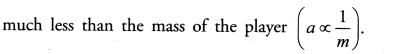(ii) When a ball falls towards the earth, the earth exerts a force (F1) on the ball to attract the ball towards its centre (figure 17).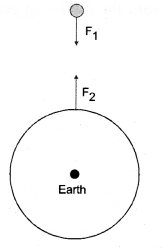On the other hand, the ball exerts a force (F2) on the earth to move the earth upward. These two forces are equal in magnitude and opposite in direction and form the action-reaction pair.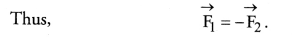The upward acceleration of earth is not noticed because the mass of the earth is very large and hence its acceleration is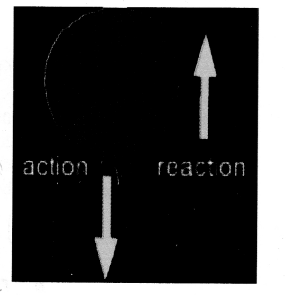From these examples, we conclude :
Whenever two bodies have influence on each other, they exert equal and opposite forces on each other. Out of these two forces, one is known as action and the other is known as reaction. Statement of Newtons third law of motion.

Question 7.
From a rifle of mass 4 kg, a bullet of mass 50 g is fired with an initial velocity of 35 m s-1. Calculate the
initial recoil velocity of the rifle. (CBSE 2011)
Before firing, both rifle and bullet are at rest. Therefore, linear momentum of the rifle and bullet before firing is zero.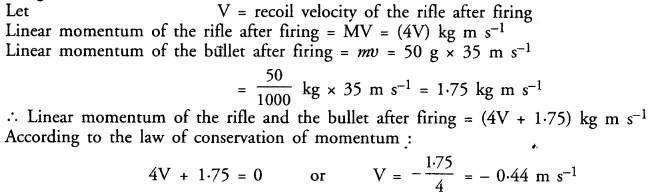Question 8.
Two objects of masses 100 g and 200 g are moving along the same line and direction with velocities of 2 m s-1 and 1 m s-1, respectively. They collide and after collision, the first object moves at a velocity of 1.67 m s-1. Determine the velocity of the second object. (CBSE 2010, 2011, 2012)
Here, mass of first object, m1= 100 g = 0.1 kg
Mass of second object, m2 = 200 g = 0.2 kg
Velocity of first object before collision, u1 = 2 m s-1
Velocity of second object before collision, u2 = 1 m s-1
Velocity of first object after collision, v1 = 1.67 m s-1
Let, velocity of second object after collision = v2
Momentum of both objects before collision =m1u1 + m2u2 = 0.1 x 2 + 0.2 x 1 = 0.4 kg m s-1
Momentum of both objects after collision = m1v1 + m2v2 = 0.1 x 1.67 + 0.2 v2 = 0.167 + 0.2 v2
According to the law of conservation of momentum :
Momentum after collision = Momentum before collision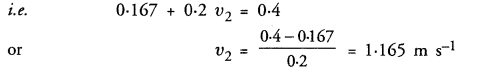NCERT CHAPTER IMP EXERCISE

Question 1.
An object experiences a net zero external unbalanced force. Is it possible for the object to be travelling with a non-zero velocity ? If yes, state the conditions that must be placed on the magnitude and direction of the velocity. If no, provide a reason. (CBSE 2010, 2011)
According to Newton’s first law of motion, no net external force is needed to move an object with constant velocity. So an object travels with a constant velocity (non-zero) when it experiences a net zero external unbalanced force. The magnitude of this velocity is constant and the direction is same as in the beginning. An object may also not move at all if it experiences a net zero external unbalanced force. This is because, the object may be at rest in the beginning.

Question 2.
When a carpet is beaten with a stick, dust comes out of it. Explain.
(CBSE 2010, 2011, 2012, 2013, 2015)
When carpet is beaten with a stick, fibre of carpet comes in motion and the dust falls down due to inertia of rest.

Question 3.
Why is it advised to tie any luggage kept on the roof of a bus with a rope ? (CBSE 2010, 2011, 2013)
When the bus suddenly stops, luggage may roll down and fall from the roof of the bus due to inertia of motion if not tied with a rope.

Question 4.
A batsman hits a cricket ball which then rolls on a level ground. After covering a short distance, the ball comes to rest. The ball slows to a stop because
(a) the batsman did not hit the ball hard enough
(b) velocity is proportional to the force exerted on the ball
(c) there is a force on the ball opposing the motion
(d) there is no unbalanced force on the ball, so the ball would want to come to rest.
(c).

Question 5.
A truck starts from rest and rolls down a hill with a constant acceleration. It travels a distance of 400 m in 20 s. Find its acceleration. Find the force acting on it if its mass is 7 metric tonnes.
(CBSE 2010, Term I, Similar CBSE 2012)
(Hint : 1 metric tonne = 1000 kg.)Question 6.
A stone of 1 kg is thrown with a velocity of 20 m s-1 across the frozen surface of a lake and comes to rest after travelling a distance of 50 m. What is the force of friction between the stone and the ice ?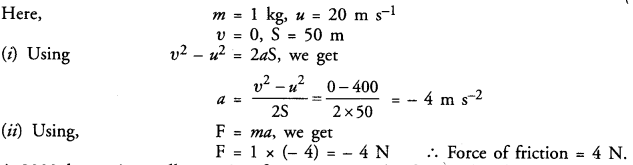Question 7.
A 8000 kg engine pulls a train of 5 wagons, each of 2000 kg along a horizontal track. If the engine exerts a force of 40,000 N and the track offers a friction force of 5000 N, then calculate :
(a) the net accelerating force
(b) the acceleration of the train. (CBSE 2010, 2011)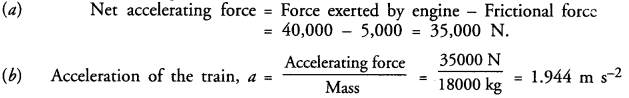Question 8.
An automobile vehicle has a mass of 1500 kg. What must be the force between the vehicle and the road if the vehicle is to be stopped with a negative acceleration of 1.7 m s-2 ?Question 9.
What is the momentum of an object of mass m, moving with velocity v ?
(a) (mv)2
(b) mv2
(c) ½ mv2
(d) mv
(d) mv.

Question 10.
Using a horizontal force of 200 N, we intend to move a wooden cabinet across a floor at a constant velocity. What is the friction force that will be exerted on the cabinet ?
The cabinet will move with constant velocity, if net external force acting on it is zero. Since a horizontal force of 200 N acts on the cabinet in the forward direction, therefore, net external force acting on it will be zero if frictional force of 200 N acts on it. Thus frictional force = 200 N will be exerted on the cabinet.

Question 11.
Two objects, each of mass 1.5 kg are moving in the same straight line but in opposite directions. The velocity of each object is 2.5 m s-1 before the collision during which they stick together. What will be the velocity of the combined object after collision
Let the two objects are A and B.
Mass of object A, m1 = 1.5 kg
Mass of object B, m2= 1.5 kg
Velocity of object A before collision, u1 = 2.5 m s-1
Velocity of object B before collision, u2 = -2.5 m s-1
Total momentum of objects A and B before collision = m1u1 + m2u2 = 1.5 x 2.5 – 1.5 x 2.5 = 0
Mass of combined object after collision = (m1 + m2) = 3.0 kg
Let, velocity of combined object after collision = V m s-1
∴ Total momentum of combined object after collision = (m1 + m2)V = (3V) kg m s-1
According to the law of conservation of momentum :
Momentum after collision = Momentum before collision 3V = 0 or V = 0

Question 12.
According to the third law of motion when we push on an object, the object pushes back on us with an equal and opposite force. If the object is a massive truck parked along the roadside, it will probably not move. A student justifies this by answering that the two opposite and equal forces cancel each other. Comment on this logic and explain why the truck does not move. (CBSE 2010, Term I)
Student’s justification is not correct. Two equal and opposite forces cancel each other if they act on the same body. According to the third law of motion, action and reaction forces are equal and opposite but they both act on different bodies. Hence, they cannot cancel each other.
When we push a massive truck, then the force applied on the truck is not sufficient to overcome the force of friction between the tyres of the truck and ground. Hence the truck does not move. The truck will move only if the force applied on it is greater than the frictional force.

Question 13.
A hockey ball of mass 200 g travelling at 10 m s-1 is struck by a hockey stick so as to return it along its
original path with a velocity at 5 m s-1 Calculate the magnitude of change of momentum occurred in the motion of the hockey ball by the force applied by the hockey stick. (CBSE 2013)
Mass of hockey ball, m = 200 g = 0.2 kg
Velocity of hockey ball before striking the hockey stick, v1 = 10 m s-1
Velocity of hockey ball after striking the hockey stick, v2 = -5 m s-1
Change of momentum = mv1 – mv2 = m(v1 – v2) = 0.2 (10 + 5) = 0.2 x 15 = 3.0 kg m s-1

Question 14.
A bullet of mass 10 g travelling horizontally with a velocity of 150 m s-1 strikes a stationary wooden block and comes to rest in 0.03 s. Calculate the distance of penetration of the bullet into the block Also calculate the magnitude of the force exerted by the wooden block on the bullet.
Mass of bullet, m = 10 g = 0.01 kg
Initial velocity of bullet, u = 150 m s-1
Final velocity of bullet, v = 0
Time taken, t = 0.03 s.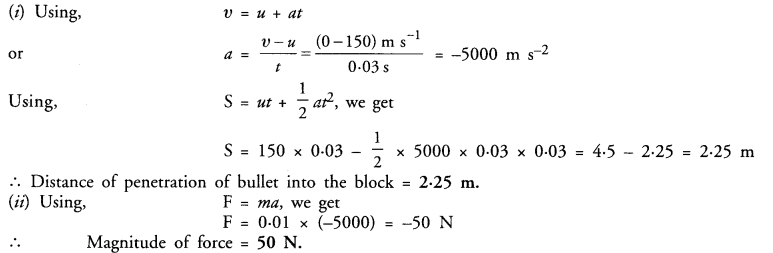Question 15.
An object of mass 1 kg travelling in a straight line with a velocity of 10 m s-1 collides with and sticks to a stationary wooden block of mass 5 kg. Then they both move off together in the same straight line. Calculate the total momentum just before the impact and just after the impact. Also, calculate the velocity of the combined object.
Mass of object, m1= 1 kg
Velocity of object before collision, u1 = 10 m s-1
Mass of wooden block, m2 = 5 kg
Velocity of wooden block before collision, u2 = 0
(i) Total momentum before the impact =m1u1 + m2u2 = 1 x 10 + 5 x 0 = 10 kg m s-1
According to the law of conservation of momentum (as no net external force acts on the system) :
Total momentum after the impact = Total momentum before the impact = 10 kg m s-1
(ii) Mass of combined object, M = mass of object + mass of block = 1 + 5 = 6 kg
Let, V = Velocity of the combined object after collision.
∴ Momentum of combined object = MV = (6V) kg m s-1
Now, momentum of combined object = Total momentum after the impact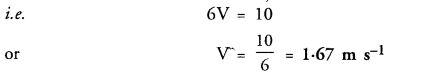Question 16.
An object of mass 100 kg is accelerated uniformly from a velocity of 5 m s-1 to 8 m s-1 in 6 s. Calculate
the initial and final momentum of the object. Also, find the magnitude of the force exerted on the object. (CBSE 2012)
Mass of object, m = 100 kg
Initial velocity, u = 5 ms-1 Final velocity, v = 8 ms-1 Time, t = 6 s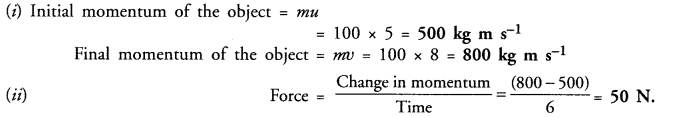Question 17.
Akhtar, Kiran and Rahul were riding in a motorcar that was moving with a high velocity on an express ‘ way when an insect hit the windshield and got stuck on the wind screen. Akhtar and Kiran started pondering over the situation. Kiran suggested that the insect suffered a greater change in momentum as compared to the change in momentum of the motorcar (because the change in the velocity of the insect was much more than that of the motorcar.) Akhtar said that since the motorcar was moving with a larger velocity, it exerted a larger force on the insect. And as a result, the insect died. Rahul while putting an entirely new explanation said that both the motorcar and the insect experienced the same force and change in their momentum. Comment on these suggestions.
Rahul was correct. The insect died because it could not bear the large force and large change in momentum.

Question 18.
How much momentum will a dumb-bell of mass 10 kg transfer to the floor if it falls from a height of 80 cm ? Take its downward acceleration to be 10 m s-1. (CBSE 2011, 2012)
Here, u = 0, m = 10 kg, S = 80 cm = 0.8 m, a = 10 m s-2
(i) Using, v2 – u2 = 2aS, we get
v2 – 0 = 2 x 10 x 0.8
or v = √16 = 4 m s-1
Momentum of the dumb-bell just before it touches the floor = mv = 10 x 4 = 40 kg m s-1
(ii) The momentum of the dumb-bell becomes zero as it touches the floor and the entire momentum is
transferred to the floor.
Momentum transferred to the floor = 40 kg m s-1.

Question 1.
The following is the distance-time table of an object in motion :

 Time in seconds 0 Distance in metres 0 1 2 3 4 5 6 7 1 8 27 64 125 216 343

(a) What conclusion can you draw about the acceleration ? Is it constant, increasing, decreasing or zero ?
(b) What do you infer about the forces acting on the object ?Time in seconds Distance in metres a = 0 0 0 1 1 2 2 8 4 3 27 6 4 64 8 5 125 10 6 216 12 7 343 14

(b) Since, acceleration is increasing, so net unbalanced force is acting on the object.

Question 2.
Two persons manage to push a motorcar of mass 1200 kg at a uniform velocity along a level road. The same motorcar can be pushed by three persons to produce an acceleration of 0.2 m s-2. With what force does each person push the motorcar ? (Assume that all persons push the motorcar with the same muscular effort.)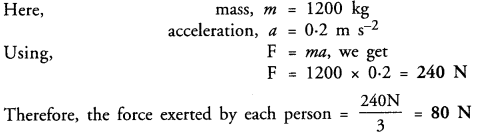Question 3.
A hammer of mass 500 g moving at 50 m s-1, strikes a nail. The nail stops the hammer in a very short time of 0.01 s. What is the force of the nail on the hammer i(CBSE 2011, 2012)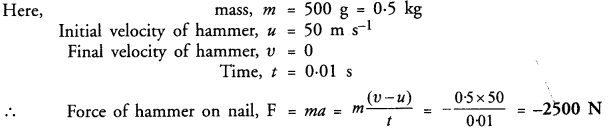Question 4.
A motor car of mass 1200 kg is moving along a straight line with a uniform velocity of 90 km/h. Its velocity is slowed down to 18 km/h in 4 s by an unbalanced external force. Calculate the acceleration and change in momentum. Also, calculate the magnitude of the force required.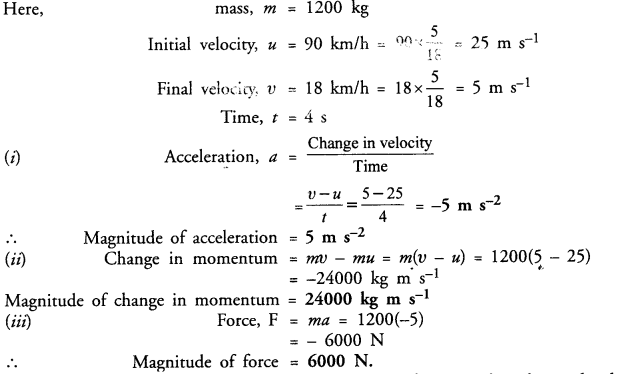Question 5.
A large truck and a car, both moving with a velocity of magnitude v, have a head-on collision and both of them come to a halt after that. If the collision lasts for 1 s :
(a) Which vehicle experiences the greater force of impact ?
(b) Which vehicle experiences the greater change in momentum ?
(c) Which vehicle experiences the greater acceleration ?
(d) Why is the car likely to suffer more damage than the truck ? (CBSE 2012)